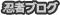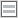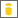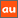# 0除算に関するアナウンスメントを公開しています

The purpose of this site is to publish announcements on the theory of division by zero

## Announcement 185 : The importance of the division by zero $z/0=0$

Institute of Reproducing Kernels
Kawauchi-cho, 5-1648-16,
Kiryu 376-0041, Japan
E-mail: kbdmm360@yahoo.co.jp

Abstract: In this announcement, we shall state the importance of the division by zero z/0=0. The result is a definite one and it is fundamental in mathematics.

1. Introduction
By a natural extension of the fractions
\begin{equation}
\frac{b}{a}
\end{equation} for any complex numbers $a$ and $b$, we, recently, found the surprising result, for any complex number $b$
\begin{equation}
\frac{b}{0}=0,
\end{equation} incidentally in  by the Tikhonov regularization for the Hadamard product inversions for matrices, and we discussed their properties and gave several physical interpretations on the general fractions in  for the case of real numbers. The result is a very special case for general fractional functions in . The division by zero has a long and mysterious story over the world $($see, for example, Google site with division by zero$)$ with its physical viewpoints since the document of zero in India on A.D. 628, however, Sin-Ei, Takahasi $($$)$ $($see also $)$ established a simple and decisive interpretation $(1.2)$ by analyzing some full extensions of fractions and by showing the complete characterization for the property $(1.2)$. His result will show that our mathematics says that the result $(1.2)$ should be accepted as a natural one:

Proposition. Let F be a function from ${\bf C }\times {\bf C }$ to ${\bf C }$ such that
\begin{equation}
F (b, a)F (c, d)= F (bc, ad)
\end{equation} for all $a, b, c, d \in {\bf C }$, and
$$F (b, a) = \frac {b}{a }, \quad a, b \in {\bf C }, a \ne 0.$$ Then, we obtain, for any $b \in {\bf C }$
$$F (b, 0) = 0.$$ Furthermore, note that Hiroshi Michiwaki gave the important interpretation of the division by zero $z/0=0$ by the intuitive meaning of the division, independently of the concept of the product $($see $)$. We shall state the importance of the division by zero $z/0=0$.

2.
On A.D. 628, the zero was appeared in India, and the zero division $z/0=0$ was discovered on February 2, 2014, definitely with the clear definition and motivation. The uniqueness and the natural interpretation were given in [7,8,4] and , respectively. Several physical interpretations of the division by zero were given in .

3.
By the introduction of the division by zero $z/0=0$, four arithmetic operations; that is, addition, subtraction, multiplication, and division are always possible; note that for division, we were not able to divide by zero. There was one exceptional case for the division by zero.

4.
For the Euclidean $($B.C. 3 Century $)$ geometry, two non-Euclidean geometries were appeared about 2 hundred years ago, and in particular, in the elliptic type non-Euclidean geometry, the point at infinity was introduced by the stereoprojection of the Euclidean plane to the sphere and the concept is a standard one in complex analysis around over one hundred years. And then we have considered as $1/0= \infty$ $($$)$. However, surprisingly enough, the division by zero means that $1/0=0$.

5.
We shall recall the fundamental law by Newton:
$F = G\frac{m_1 m_2}{r^2} \tag{5.1}$ for two masses $m_1, m_2$ with a distance $r$ and a constant $G$. Of course,
$\lim_{r \to +0} F =\infty,$ however, we obtain the important interpretation:
$F = 0 = G \frac{m_1 m_2}{0}. \tag{5.2}$ Of course, here, we can consider the above interpretation for the mathematical formula $($5.1$)$ as the new interpretation $($5.2$)$. We can find many physical formulas with the division by zero.

6.
In complex analysis, linear fractional functions
$$W = \frac{az + b}{cz + d}, \quad ad -bc \ne 0,$$ map the extended complex plane onto the extended complex plane containing the point at infinity, one to one, conformally, beautifully. This beautiful property is changed as the beautiful formula that linear fractional functions map the whole complex plane onto the whole complex plane, one to one, however, at one point of the singular point, the linear fractional functions have strong discontinuity. The division by zero excludes the infinity from the numbers.

7.
We did, essentially, not consider the division by zero, and so the property of the division by zero; that is, at the isolated singular points of analytic functions, to consider the analytic functions are new mathematics and new research topics, essentially.

8.
The impact to complex analysis is unclear, we, however, obtain a typical new theorem:
Theorem : Any analytic function takes a definite value at an isolated singular point with a natural meaning. The definite value is given by the first coefficient of the regular part in the Laurent expansion around the isolated singular point.

This will be the fundamental theorem on the division by zero in Complex Analysis and we have many applications for the Sato hyperfunction theory, generating functions theory and singular integral theory $($$)$.

9.
In particular, the division by zero gives new interpretations on the finite part of Hadamard
for singular integrals and the Cauchy's principal values. The division by zero will represent discontinuity properties on the universe.

10.
Even for middle high school students, the division by zero may be accepted as the beautiful result with great pleasures: For the elementary function
$$y = f(x) = \frac{1}{x},$$ we have $f(0) = 0$; that is, $1/0=0$.

11.
We can introduce the division by zero $100/0=0,0/0=0$ with the simple and natural definition for the division by the Hiroshi Michiwaki method $($$)$ in the elementary school. The division by zero will request the change of all the related books and scientific books.

12. Conclusion
The division by zero $b/0=0$ is possible and the result is naturally determined, uniquely.
The result does not contradict with the present mathematics - however, in complex analysis, we need only to change a little presentation for the pole; not essentially, because we did not consider the division by zero, essentially. The common understanding that the division by zero is impossible should be changed with many text books and mathematical science books. The definition of the fractions may be introduced by it the method of Michiwaki in the elementary school, even. Should we teach the beautiful fact, widely?: For the elementary graph of the fundamental function
$$y = f(x) = \frac{1}{x},$$ $$f(0) = 0.$$ The result is applicable widely and will give a new understanding for the universe $($ Announcement 166$)$.

If the division by zero $b/0=0$ is not introduced, then it seems that mathematics is incomplete in a sense, and by the introduction of the division by zero, mathematics will become complete in a sense and perfectly beautiful.

Remarks
For the procedure of the developing of the division by zero and for some general ideas on the division by zero, we presented the following announcements in Japanese:
Announcement 148 $($2014.2.12$)$: $100/0=0, 0/0=0$ -- by a natural extension of fractions -- A wish of the God.
Announcement 154 $($2014.4.22$)$: A new world: division by zero, a curious world, a new idea.
Announcement 157 $($2014.5.8$)$: We wish to know the idea of the God for the division by zero; why the infinity and zero point are coincident?
Announcement 161 $($2014.5.30$)$: Learning from the division by zero, spirit of mathematics and of looking for the truth.
Announcement 163 $($2014.6.17$)$: The division by zero, an extremely pleasant mathematics - shall we look for the pleasant division by zero: a proposal for a fun club looking for the division by zero.
Announcement 166 $($2014.6.29$)$: New general ideas for the universe from the viewpoint of the division by zero.
Announcement 171 $($2014.7.30$)$: The meanings of product and division--The division by zero is trivial from the own sense of the division independently of the concept of product
Announcement 176 $($2014.8.9$)$: Should be changed the education of the division by zero.
Announcement 179 $($2014.10.22$)$: Division by zero is clear as z/0=0 and it is fundamental in mathematics.

References
 L. V. Ahlfors, Complex Analysis, McGraw-Hill Book Company, 1966.
 L. P. Castro and S.Saitoh, Fractional functions and their representations, Complex Anal. Oper. Theory Vol. 7 $($2013$)$, no. 4, 1049-1063.
 S. Koshiba, H. Michiwaki, S. Saitoh and M. Yamane, An interpretation of the division by zero z/0=0 without the concept of product, $($note$)$.
 M. Kuroda, H. Michiwaki, S. Saitoh, and M. Yamane,
New meanings of the division by zero and interpretations on $100/0=0$ and on $0/0=0$,
Int. J. Appl. Math. Vol. 27, No 2 $($2014$)$, pp. 191-198, DOI: 10.12732 ijam.v27i2.9.
 H. Michiwaki, S. Saitoh, and M. Takagi, A new concept for the point at infinity and the division by zero $z/0=0$ $($note$)$.
 S. Saitoh, Generalized inversions of Hadamard and tensor products for matrices, Advances in Linear Algebra & Matrix Theory. Vol.4 No.2 $($2014$)$, 87-95. http://www.scirp.org/journal/ALAMT/
 S.-E. Takahasi, On the identities $100/0=0$ and $0/0=0$, $($note$)$.
 S.-E. Takahasi, M. Tsukada and Y. Kobayashi, Classification of continuous fractional binary operators on the real and complex fields, Tokyo Journal of Mathematics $($in press$)$.
 Announcement 179: Division by zero is clear as $z/0=0$ and it is fundamental in mathematics, Institute of Reproducing Kernels, 2014.10.22. $($2013$)$, no. 4, 1049-1063.

PR

### コメント

お名前 Black LimeGreen SeaGreen Teal FireBrick Tomato IndianRed BurlyWood SlateGray DarkSlateBlue LightPink DeepPink DarkOrange Gold DimGray Silverプロフィール

ＨＮ：

(01/17)
(01/14)
(01/12)
(01/04)
(01/03)